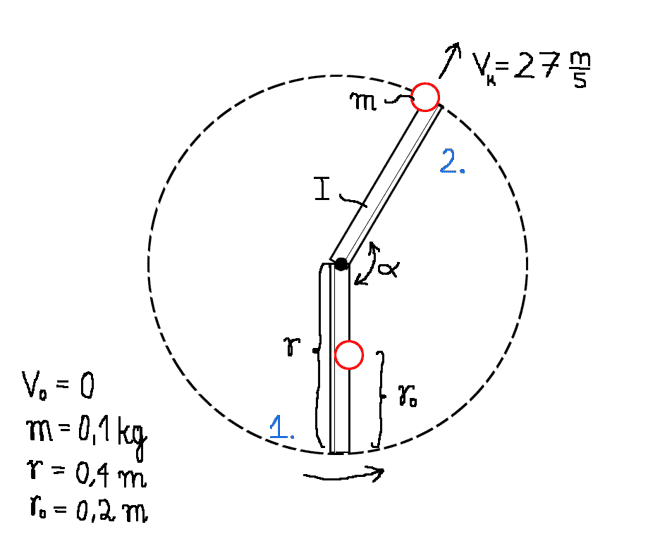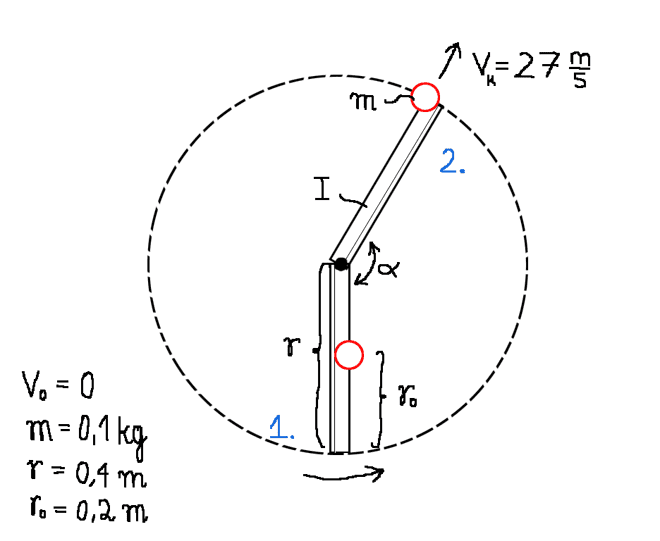# Throwing force in target thrower

Hi! I need a help in desiging a target thrower. I can't find a force that is needed in order to throw a target with certain velocity. I assume that it's gonna be a centrifugal force, but I can't find a connection with linear movement of target and rotation of an arm. Does anyone have an idea? Here's a picure:DaveC426913
Gold Member
What is r0? I see it in the diagram, but what is it doing there? Is there more than one moving component?

berkeman
Mentor
Hi! I need a help in desiging a target thrower. I can't find a force that is needed in order to throw a target with certain velocity. I assume that it's gonna be a centrifugal force, but I can't find a connection with linear movement of target and rotation of an arm. Does anyone have an idea? Here's a picure:Welcome to the PF.

Is this for launching clay "pigeons" like in trap shooting? Is it a flat disk or a round ball that you are launching. If it's a flat disk like a clay pigeon, you will want it to roll a ways down the launch blade as the blade is going through its motion. That will give it the spin it needs to fly stably.

Is this a spring-powered launcher? If so, you may be able to do a pretty simple calculation equating the energy stored in the spring when cocked with the final energy of the moving arm and the moving target (linear velocity and energy stored in its rotation)...

What is r0? I see it in the diagram, but what is it doing there? Is there more than one moving component?

r0 is a path, that disc has to travel, from it's initial position (V0=0), to the moment when it leaves the arm (V=27)

Welcome to the PF.

Is this for launching clay "pigeons" like in trap shooting? Is it a flat disk or a round ball that you are launching. If it's a flat disk like a clay pigeon, you will want it to roll a ways down the launch blade as the blade is going through its motion. That will give it the spin it needs to fly stably.

Is this a spring-powered launcher? If so, you may be able to do a pretty simple calculation equating the energy stored in the spring when cocked with the final energy of the moving arm and the moving target (linear velocity and energy stored in its rotation)...

Yes, it is a clay launcher/thrower. It is spring-powered. I was thinking about the energy calculations, but I thought that it would leave the aspect of WHEN the target leaves the arm. I want it to leave, when the arm travels about 160°-170°. In energy equation I need also ω of the arm and that's the problem. I can't find proper ω of the arm.

DaveC426913
Gold Member
r0 is a path, that disc has to travel, from it's initial position (V0=0), to the moment when it leaves the arm (V=27)
Ohhhh I see. So it follows a spiral path.

Ohhhh I see. So it follows a spiral path.

Yes, exactly! I'm using that equation: E=1/2*(m*V^2 + Iω^2 + Iω^2). I know V and ω of disc, but I don't know how to calculate ω of the arm.

berkeman
Mentor
Have you seen how basic clay pigeon launchers work? You tune the launch angle by moving the clay pigeon forward and back in a friction hold channel...

Have you seen how basic clay pigeon launchers work? You tune the launch angle by moving the clay pigeon forward and back in a friction hold channel...

Yes, I know, but I have to calculate it somehow (ω of the arm), because I need it to equation of energy.

berkeman
Mentor
For the launchers I've used, it's pretty empirical. Why do you need a full mathematical solution? Are you going to hold the friction holding channel on the launcher to tight tolerances? And the clay pigeons? What accuracy are you shooting for (pun) in the launch angle?

For the launchers I've used, it's pretty empirical. Why do you need a full mathematical solution? Are you going to hold the friction holding channel on the launcher to tight tolerances? And the clay pigeons? What accuracy are you shooting for (pun) in the launch angle?

Well, maybe not full solution. The thing is I need to choose proper spring. I will do it from energy equation, just like You sugested. To do so, I need angular and linear velocity of clay target (which I have) and angular velocity of the arm. And that's the problem, how to calculate ω of the arm. I can't find connection between ω of arm and velocities of clay target. Not to mention, that the friction channel is complicating the case even more, cuz I don't have an idea how to take it to account neither.

Please Kamil, I don't understand well how the mechanism works. I was trying to know the mechanism at Internet, but I didn't find an scheme of it.If you draw the two positions, the initial and the final position, of the mechanism bars, I think it woul be possible to find out the relations you are looking for.

DaveC426913
Gold Member
BTW, I know it's just a sketch but I'm pretty sure your exit trajectory is way off. The clay pigeon's trajectory will form a parabola, transformed into polar coordinates. So its exit from the launcher should look more like this:

#### Attachments

•berkeman
Thanks Dave, I was thinking in other system, and I see the sketch shows the two position.
Kamil, I have not found an easy solution: two second order nonlinear differential equations, which, of course, there would be possible to solve numericaly .
x is the distance target-center of the bar
v es the speed of the target which is the vectorial sum of the two ortogonal vectors: \dot{x} and \dot{α}.x
k is the spring constant

The two differential equations are:

(1). \ddot{α}.(I+m.x)=k.α

(2). \ddot{x}=\dotα2.x

Sorry i don't know how to write the equations properly. I'll try to solve it.

Last edited:
Whoa! Thank You guys for so many responces! I made some calculations and it came out, that ω of the arm equals 63,5 [rad/s]. Angle, for which target will leave arm, equals about 60°. You think these are pretty real results? Considering Dave's graph, I think that's a good angle.